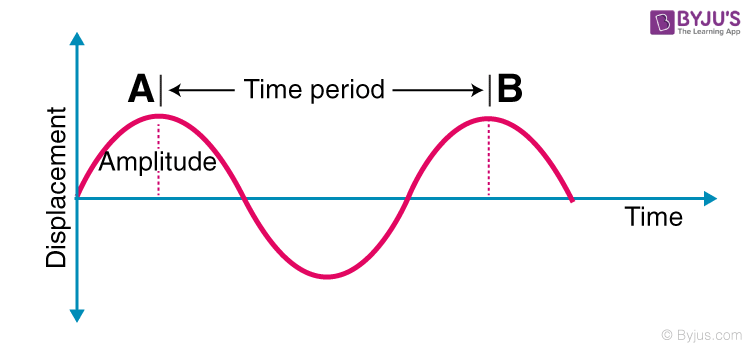Checkout JEE MAINS 2022 Question Paper Analysis : Checkout JEE MAINS 2022 Question Paper Analysis :

# Introduction To Periodic Motion

We can classify the motion of various bodies on the basis of the way they move. For example, a car moving on a straight road is said to have linear motion. Similarly, the motion of the earth around the sun is circular motion. In this session, we shall be discussing periodic motion along with its formula.

## Periodic Motion

A motion that repeats itself after equal intervals of time is known as periodic motion.
Examples of periodic motion: a tuning fork or motion of a pendulum if you analyze the motion you will find that the pendulum passes through the mean position only after a definite interval of time. We can also classify the above motion to be oscillatory. An oscillatory is a motion in which the body moves to and fro about a fixed position. So an oscillatory motion can be periodic but it is not necessary.
So taking an example of a wave motion we will see some parameters related to periodic motion. Let’s take the following figure:## Periodic Motion Formula

Time Period (T): It is the time taken by the motion to repeat itself. So the unit of a time period is seconds.
Frequency (f): It is defined as a number of times the motion is repeated in one second. The unit of frequency is Hz (Hertz). Frequency is related to Time period as:

 $$\begin{array}{l}f=\frac{1}{T}\end{array}$$

Above is the formula used for periodic motion.
To know more about motion, see the video below.## Simple Harmonic Motion

We also have heard of something known as simple harmonic motion. What is a simple harmonic motion? It is actually a periodic motion having some relation between the restoring force and displacement from the mean position. SHM is a special case of oscillation in which motion takes place along a straight line between the two extreme points. A restoring force is seen directed towards the mean position or towards the equilibrium position in simple harmonic motion. The mean position in simple harmonic motion is a stable equilibrium.

To know about simple harmonic motion in detail, see the video below.Hope you have understood in brief the periodic motion, periodic motion formula, and simple harmonic motion. Stay tuned with BYJU’S to learn more on Physics-related concepts.

## Frequently Asked Questions – FAQs

### What is periodic motion?

A motion that repeats itself after equal intervals of time is known as periodic motion.

### What is a simple harmonic motion?

A motion in which the restoring force is directly proportional to the displacement of the body from its mean position is known as Simple Harmonic Motion (SHM).

### What is the unit of frequency?

Unit of frequency is Hertz (Hz).

### Give the formula to find the frequency.

The frequency is given by the formula, f=1/T.
Where, f=frequency and T = Time Period.

### What is circular motion?

Movement of an object while rotating along a circular path is known as circular motion.
Test your Knowledge on Periodic Motion